# foldr CS 5010 Program Design Paradigms Bootcamp Lesson

• Slides: 26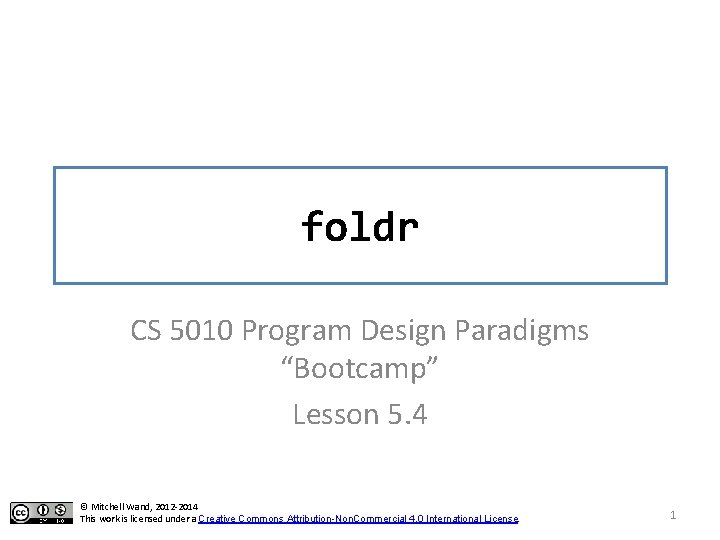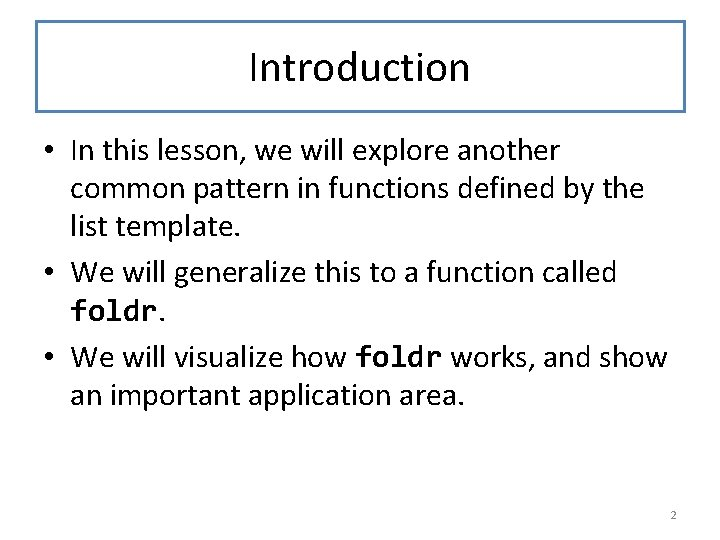Introduction • In this lesson, we will explore another common pattern in functions defined by the list template. • We will generalize this to a function called foldr. • We will visualize how foldr works, and show an important application area. 2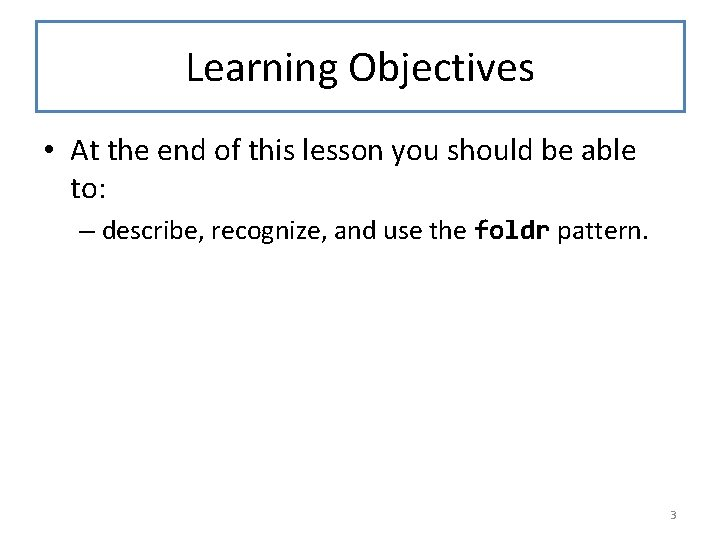Learning Objectives • At the end of this lesson you should be able to: – describe, recognize, and use the foldr pattern. 3What else could be different? ; List. Of. Number -> List. Of. Number (define (add-1 -to-each lon) (cond [(empty? lon) empty] [(else (cons (add 1 (first lon)) (add 1 -to-each (rest lon))))])) ; List. Of. Employee -> List. Of. String (define (extract-names lop) (cond [(empty? lop) empty] [else (cons (Employee-name (first lop)) (extract-names (rest lop)))])) Here is the example we used to introduce map. In this example, both of the brown functions are cons, but in some other function there could be something else in that position. 4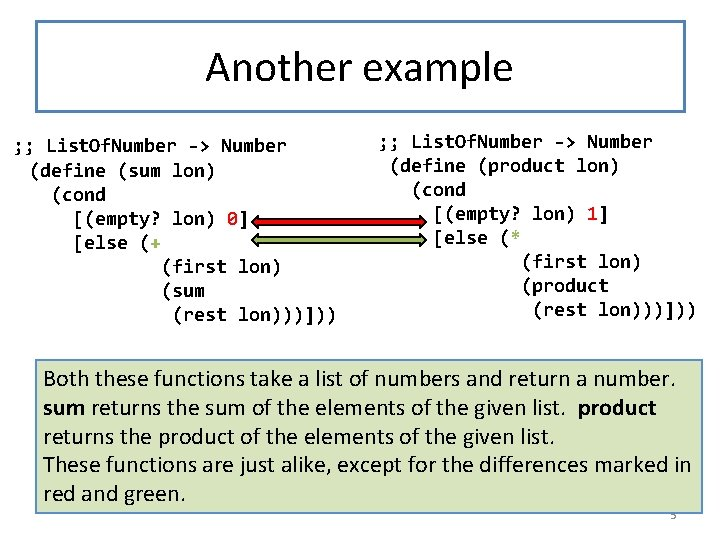Another example ; ; List. Of. Number -> Number (define (sum lon) (cond [(empty? lon) 0] [else (+ (first lon) (sum (rest lon)))])) ; ; List. Of. Number -> Number (define (product lon) (cond [(empty? lon) 1] [else (* (first lon) (product (rest lon)))])) Both these functions take a list of numbers and return a number. sum returns the sum of the elements of the given list. product returns the product of the elements of the given list. These functions are just alike, except for the differences marked in red and green. 5Let's generalize these • sum and product can be generalized to a function we call foldr, with two new arguments: one called fcn, for the function in the green position, and one called val, for the value in the red position. The strategy for foldr is using the template for List. Of. X on its list argument. • Our original sum and product functions can be recreated by supplying + and 0, or * and 1, as the two arguments. The strategy for these new versions of sum and product is "Use HOF foldr". • The name foldr is a standard name for this function, so that is the name we will use. foldr is already defined in ISL, so you don't need to write out the definition. • Let's look at the code: 6Create two new arguments for the two differences. We call this "foldr" (we'll explain the name later) (define (foldr fcn val lon) This is predefined in ISL, so (cond you don't need to write out this definition [(empty? lon) val] [else (fcn (first lon) (foldr fcn val (rest lon)))])) ; ; strategy: Use HOF foldr on lon (define (sum lon) (foldr + 0 lon)) (define (product lon) (foldr * 1 lon)) 7What is the purpose statement? ; ; foldr : (X Y -> Y) Y List. Of. X -> Y ; ; RETURNS: the result of applying f on the ; ; elements of the given list ; ; from right to left, starting with base. ; ; (foldr f base (list x_1. . . x_n)) ; ; = (f x_1. . . (f x_n base)) 8What is the contract for foldr? Based on our two examples we might guess the following contract for foldr: Here is one guess for the contract for foldr, based on our two examples: foldr : (Number -> Number) Number List. Of. Number -> Number This works, because + and * both have contract (Number -> Number), and 0 and 1 are both numbers. 9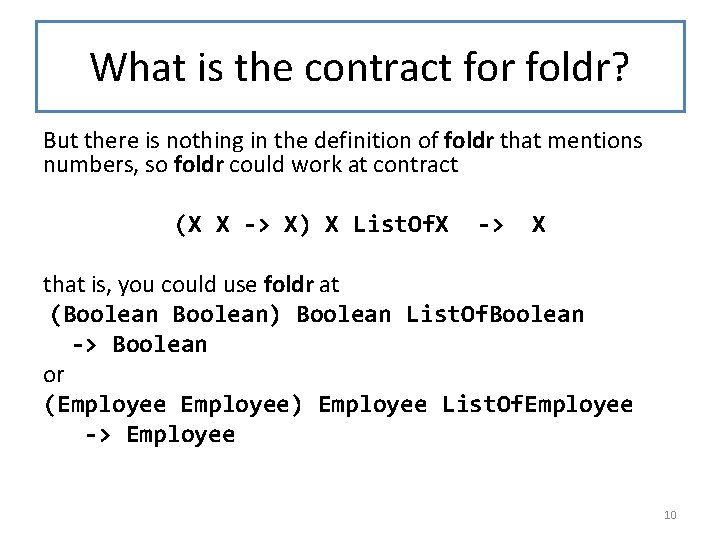What is the contract for foldr? But there is nothing in the definition of foldr that mentions numbers, so foldr could work at contract (X X -> X) X List. Of. X -> X that is, you could use foldr at (Boolean) Boolean List. Of. Boolean -> Boolean or (Employee) Employee List. Of. Employee -> Employee 10Let's watch foldr compute on this list y 4 fcn x 4 x 3 y 3 fcn x 4 x 1 empty y 1 fcn x 2 y 2 fcn x 1 val Step through the animation to watch the computation of (foldr fcn val (list x 4 x 3 x 2 x 1)) 11What can we learn from this? • The base value val is a possible 2 nd argument to fcn. • The result of fcn becomes a 2 nd argument to fcn. • So this will work as long as – val, – the 2 nd argument to fcn, – and the result of fcn are all of the same type. • So fcn must satisfy the contract (X Y -> Y) for some X and Y. 12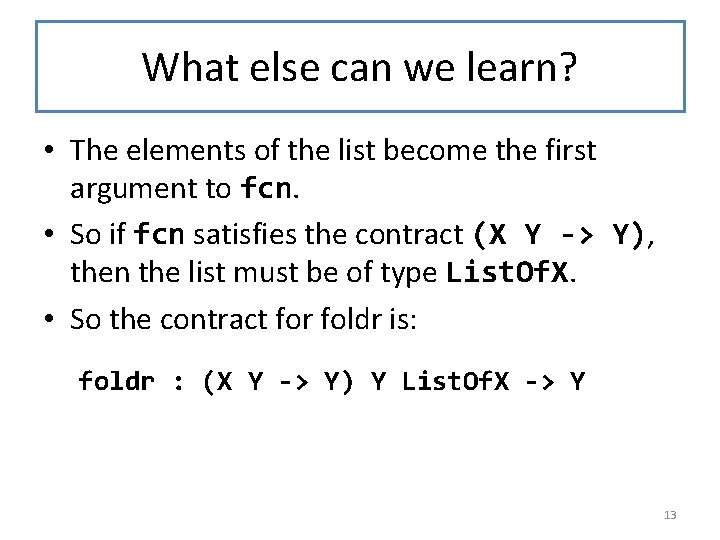What else can we learn? • The elements of the list become the first argument to fcn. • So if fcn satisfies the contract (X Y -> Y), then the list must be of type List. Of. X. • So the contract for foldr is: foldr : (X Y -> Y) Y List. Of. X -> Y 13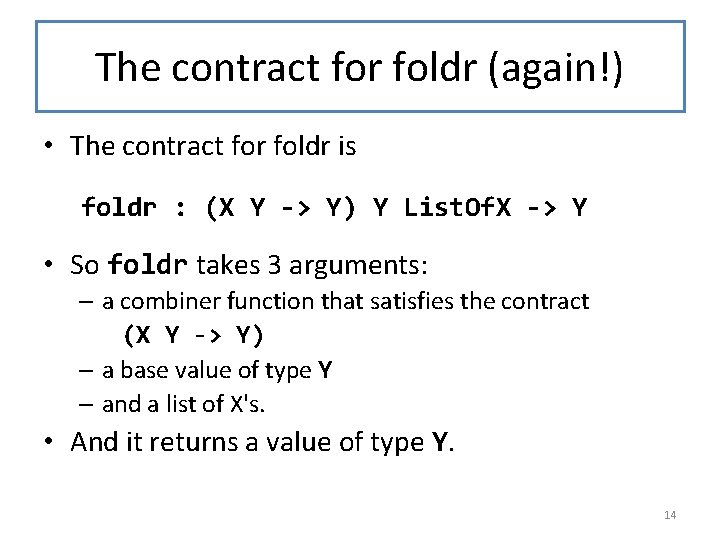The contract for foldr (again!) • The contract for foldr is foldr : (X Y -> Y) Y List. Of. X -> Y • So foldr takes 3 arguments: – a combiner function that satisfies the contract (X Y -> Y) – a base value of type Y – and a list of X's. • And it returns a value of type Y. 14Another picture of foldr Here's another visualization of foldr that you may find helpful. ( x 1 x 2 x 3 x 4 x 5 ) f f f val (foldr f val (list x 1. . . x 5)) 15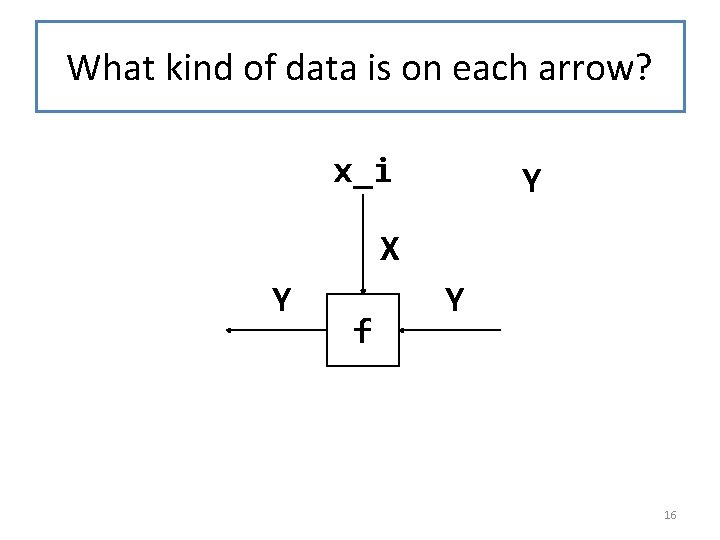What kind of data is on each arrow? x_i Y X Y f Y 16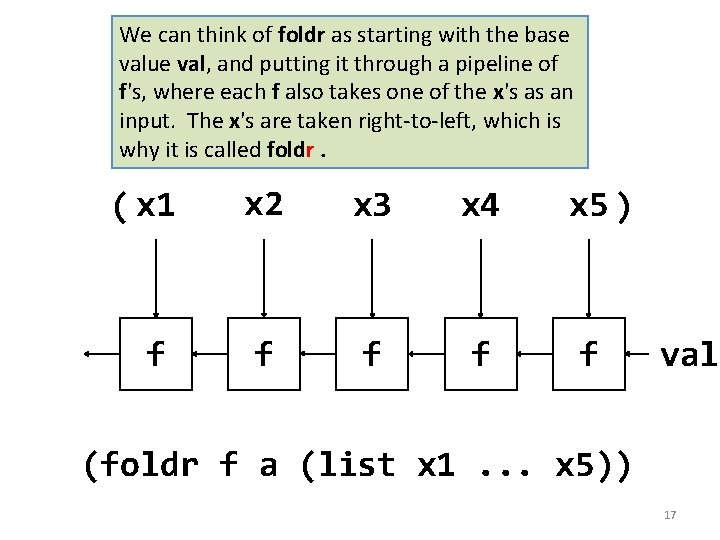We can think of foldr as starting with the base value val, and putting it through a pipeline of f's, where each f also takes one of the x's as an input. The x's are taken right-to-left, which is why it is called foldr. ( x 1 x 2 x 3 x 4 x 5 ) f f f val (foldr f a (list x 1. . . x 5)) 17Another example: ; ; strategy: combine simpler functions What is the contract for (define (add 1 -if-true b n) (if b (+ n 1) n))) add 1 -if-true ? At what ; ; strategy: Use HOF foldr on lob (define (count-trues lob) (foldr add 1 -if-true 0 lob)) Or even better: ; ; strategy: Use HOF foldr on lob (define (count-trues lob) (local ((define (add 1 -if-true b n) (if b (+ n 1) n))) (foldr add 1 -if-true 0 lob))) contract is foldr being used in this example? What is returned by count -trues ? Try to answer these questions before proceeding to the next slide. 18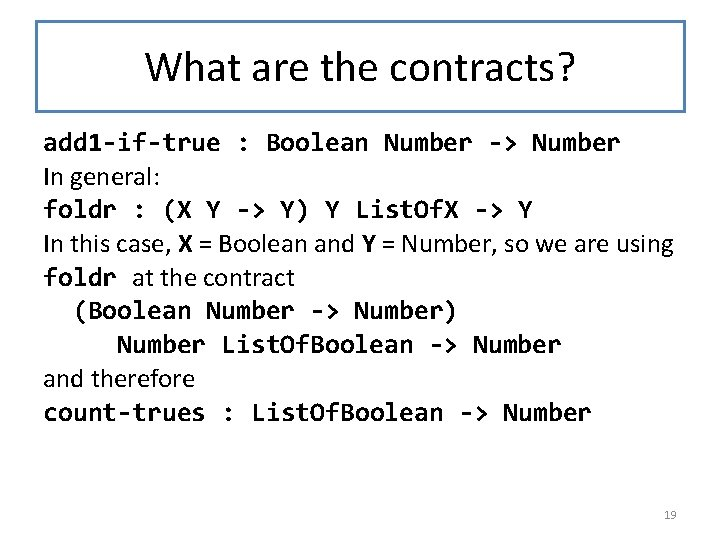What are the contracts? add 1 -if-true : Boolean Number -> Number In general: foldr : (X Y -> Y) Y List. Of. X -> Y In this case, X = Boolean and Y = Number, so we are using foldr at the contract (Boolean Number -> Number) Number List. Of. Boolean -> Number and therefore count-trues : List. Of. Boolean -> Number 19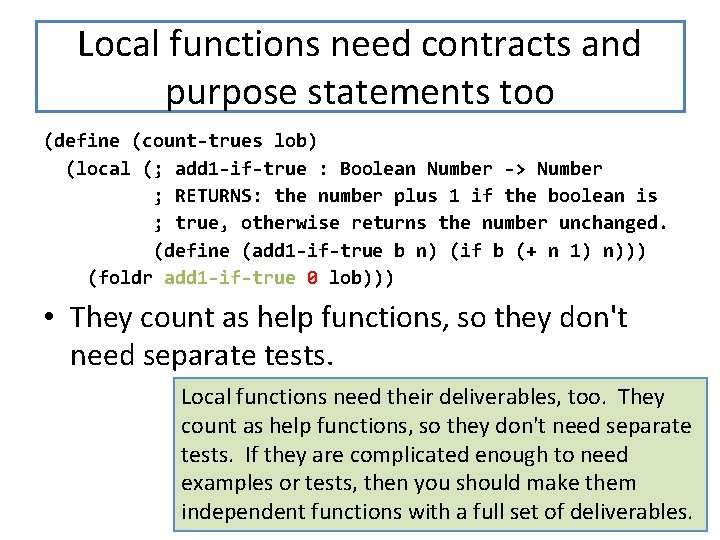Local functions need contracts and purpose statements too (define (count-trues lob) (local (; add 1 -if-true : Boolean Number -> Number ; RETURNS: the number plus 1 if the boolean is ; true, otherwise returns the number unchanged. (define (add 1 -if-true b n) (if b (+ n 1) n))) (foldr add 1 -if-true 0 lob))) • They count as help functions, so they don't need separate tests. Local functions need their deliverables, too. They count as help functions, so they don't need separate tests. If they are complicated enough to need examples or tests, then you should make them 20 independent functions with a full set of deliverables.The whole thing (less examples and tests) ; ; count-trues : List. Of. Boolean -> Number ; ; RETURNS: the number of trues in the given list of booleans. ; ; STRATEGY: Use HOF foldr on lob (define (count-trues lob) (local (; add 1 -if-true : Boolean Number -> Number ; RETURNS: the number plus 1 if the boolean is ; true, otherwise returns the number unchanged. (define (add 1 -if-true b n) (if b (+ n 1) n))) (foldr add 1 -if-true 0 lob))) 21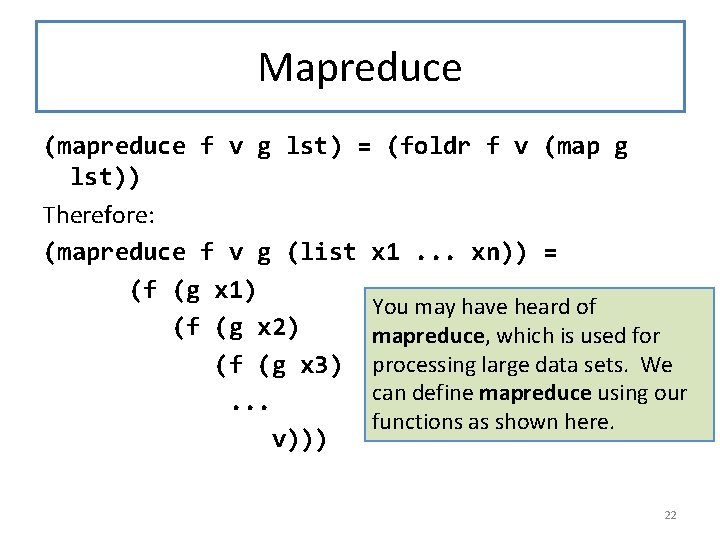Mapreduce (mapreduce f v g lst) = (foldr f v (map g lst)) Therefore: (mapreduce f v g (list x 1. . . xn)) = (f (g x 1) You may have heard of (f (g x 2) mapreduce, which is used for (f (g x 3) processing large data sets. We can define mapreduce using our . . . functions as shown here. v))) 22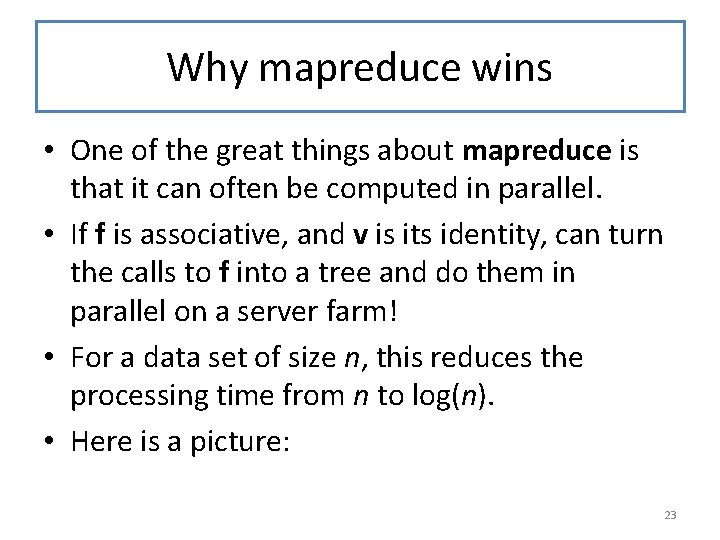Why mapreduce wins • One of the great things about mapreduce is that it can often be computed in parallel. • If f is associative, and v is its identity, can turn the calls to f into a tree and do them in parallel on a server farm! • For a data set of size n, this reduces the processing time from n to log(n). • Here is a picture: 23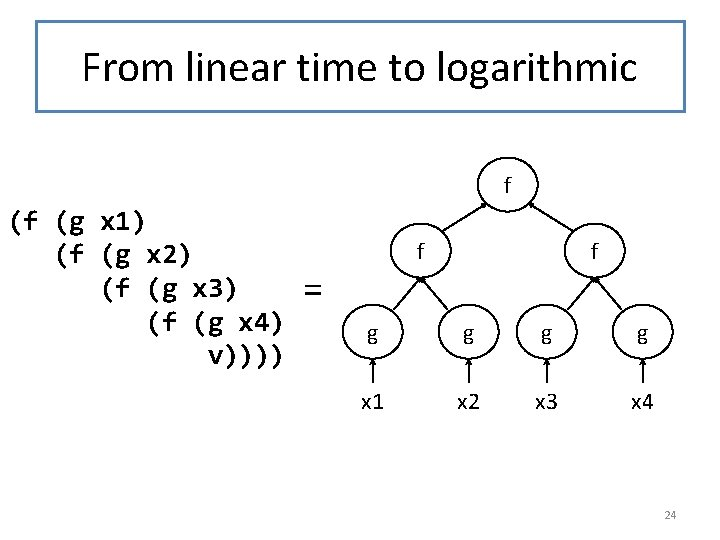From linear time to logarithmic f (f (g x 1) (f (g x 2) (f (g x 3) (f (g x 4) v)))) f = f g g x 1 x 2 x 3 x 4 24Summary • You should now be able to: – describe, recognize, and use the foldr pattern. – state the contracts for ormap, and filter and foldr, and use them appropriately. – combine these functions using higher-order function combination. 25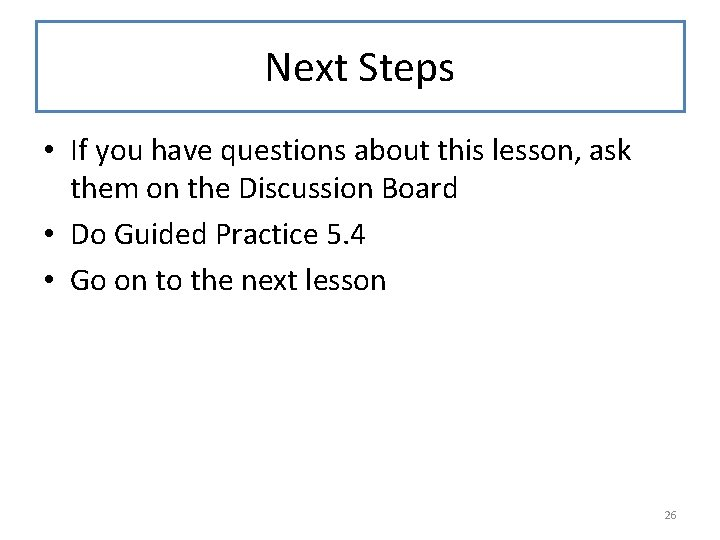Next Steps • If you have questions about this lesson, ask them on the Discussion Board • Do Guided Practice 5. 4 • Go on to the next lesson 26